# How to Convert Formula to Text String in Excel?

Generally, in Excel, when we type "=" before any string, it will be treated as a formula. But sometimes there can be some strings that are needed to be entered on a sheet that start with the "=" sign. And also, sometimes we needed to convert the existing formulas to strings.

In this tutorial, we will learn how to convert formulas to text strings in Excel. We can complete this process by using the find and replace function in Excel. In this process, all the formulas present in the sheet will be converted into strings.

## Converting a Formula to Text String in Excel

Here we will insert a space before the equal symbol to complete the task. Let's take a look at a simple procedure for converting formulas to text strings in Excel. We can complete it by using the find and replace function in Excel.

### Step 1

Let us consider any Excel sheet where the data in the sheet is similar to the below image.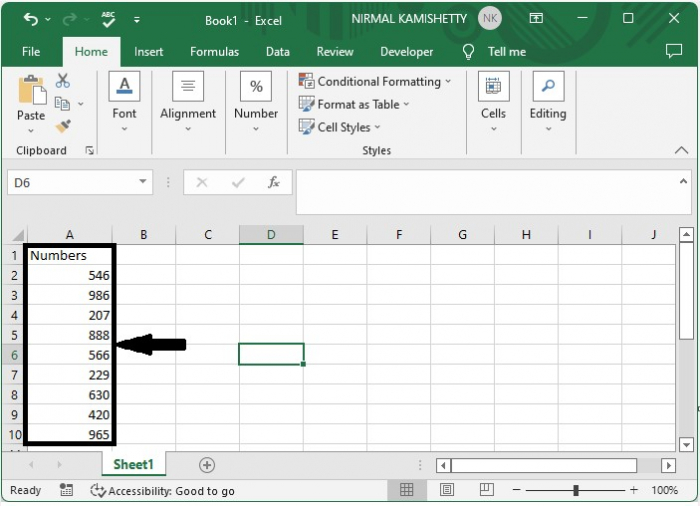To apply the formula Click on any empty cell, in our case cell B2, and enter the formula as "=A2/2" and click Enter, as shown in the below image.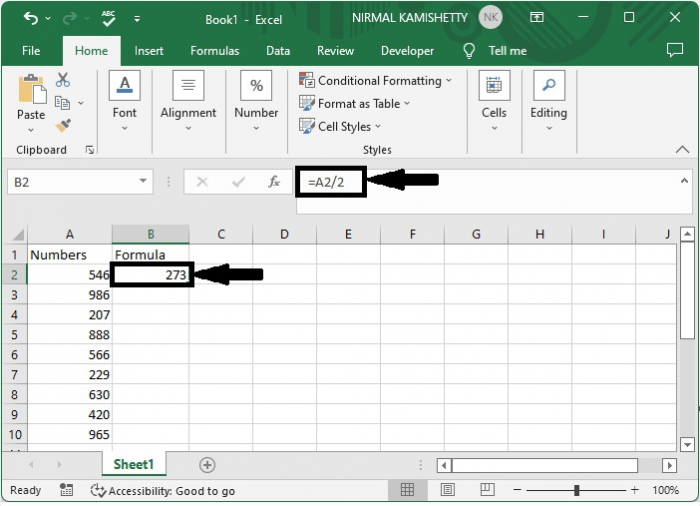### Step 2

To apply the formula to all the cells drag down from the first result using the auto fill handle and it will look similar to screenshot given below.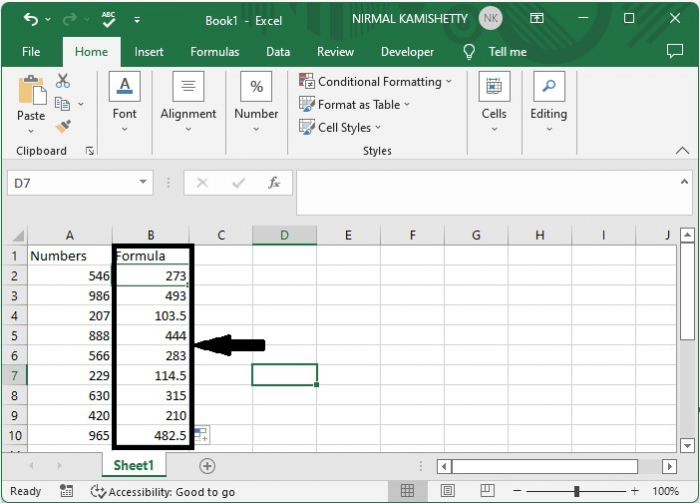### Step 3

To convert the formula to a string, use the command CTRL + F to open the find and replace menu, then click on replace, enter "=" in find what and " =" in replace with, and click on replace all as shown in the below image.

CTRL + F > Replace > "=" > " =" > Replace all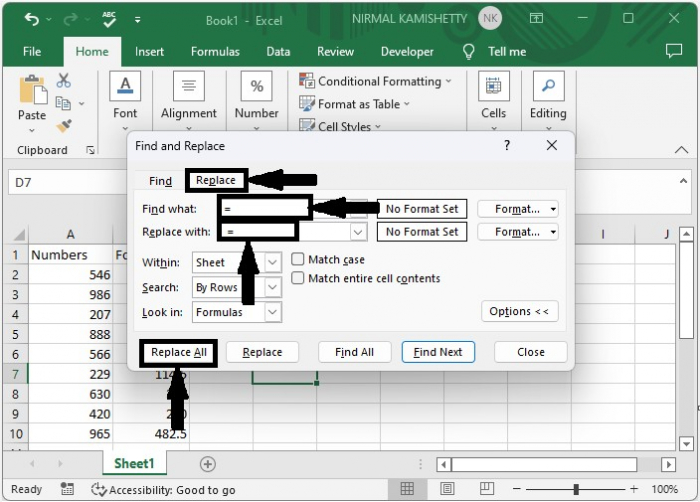And our final output will look similar to the screenshot given below.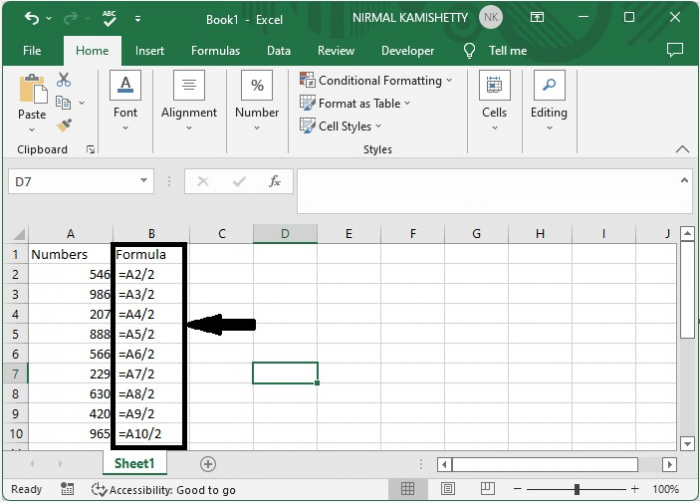## Conclusion

In this tutorial, we used a simple example to demonstrate how you can convert a formula to a text string in Excel.

Updated on: 08-Feb-2023

8K+ Views